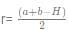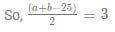A right angled triangle has an inradius of length 3 cm and a circumradius of length 12.5 cm. What is the area of the triangle in square cms?

### Related TestPathways Academy Feb 12, 2022We know that circumradius, R = half of hypotenuse.
∴ Hypotenuse H = 25 cm.
Also, inradiuswhere a and b are the perpendicular sides of the right angled triangle.⇒ a + b = 31. ....(1)
Also, a+ b2 = H2
⇒ a2 + b2 = 625 .....(2)
Squaring (1) and subtracting (2) from it, we get:
a2 + b2 + 2ab - a- b2 = 961 - 625
⇒ 2ab = 336
ab = 168
Area of a right angled triangle = ab/2 = 168/2 = 84 sq. cms

We know that circumradius, R = half of hypotenuse.∴ Hypotenuse H = 25 cm.Also, inradiuswhere a and b are the perpendicular sides of the right angled triangle.⇒ a + b = 31. ....(1)Also, a2+ b2 = H2⇒ a2 + b2 = 625 .....(2)Squaring (1) and subtracting (2) from it, we get:a2 + b2 + 2ab - a2- b2 = 961 - 625⇒ 2ab = 336ab = 168Area of a right angled triangle = ab/2 = 168/2 = 84 sq. cms
Do you know? How Toppers prepare for CAT Exam
With help of the best CAT teachers & toppers, We have prepared a guide for student who are preparing for CAT : 15 Steps to clear CAT Exam